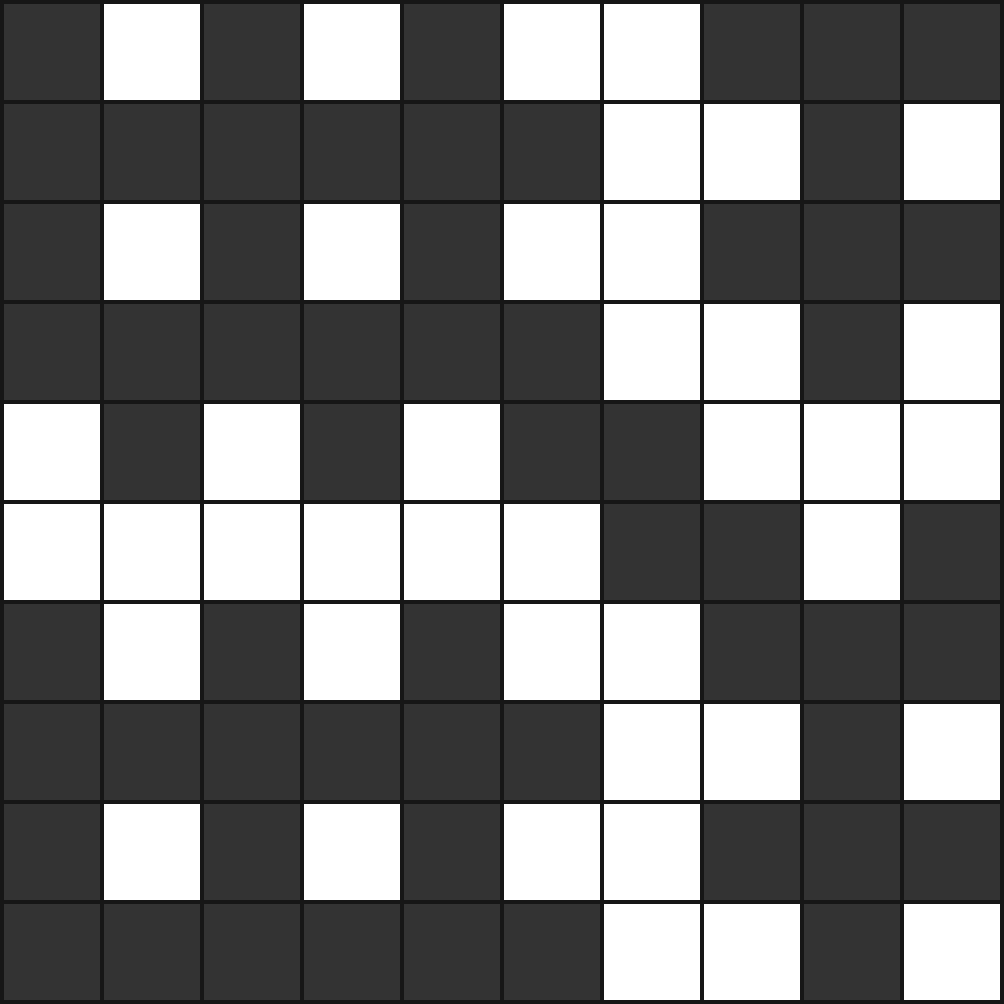# Chessboard Colouring

Probability Level 3

How many different colorings of a $10 \times 10$ square grid are there such that the following 2 conditions are met?

1. Each square must be either black or white.
2. Each $2 \times 2$ square in the grid must contain an odd number of $1 \times 1$ black squares.An example of how the grid can be colored

×